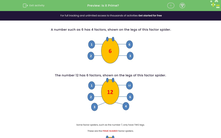# Recognise Prime Numbers

In this worksheet, students will state whether or not a number is prime.Key stage:  KS 2

Curriculum topic:   Number: Multiplication and Division

Curriculum subtopic:   Know Prime Numbers

Popular topics:   Prime Numbers worksheets, Factors worksheets

Difficulty level:#### Worksheet Overview

In this activity, we are going to be looking at the factors of numbers.

The number 6 has four factors, shown in pairs on the legs of this factor spider.

The factor pairs of 6 are:

1 and 6

2 and 3

So, it has two factor pairs and four factors: 1, 2, 3 and 6The number 12 has six factors, shown on the legs of this factor spider.The factor pairs of 12 are:
1 and 12
2 and 6
3 and 4

So there are three factor pairs and six factors: 1, 2, 3, 4, 6 and 12

Some factor spiders, such as the number 7, only have two legs.

These are the prime number factor spiders.Prime numbers are very special.

They have only two factors: 1 and the number itself.

Let's have a go at some questions now.

### What is EdPlace?

We're your National Curriculum aligned online education content provider helping each child succeed in English, maths and science from year 1 to GCSE. With an EdPlace account you’ll be able to track and measure progress, helping each child achieve their best. We build confidence and attainment by personalising each child’s learning at a level that suits them.

Get started